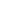#GED® Exam Prep: Mathematical Reasoning is a Course

## GED® Exam Prep: Mathematical Reasoning

Time limit: 180 days

### Full course description

This completely online and self-paced, GED® Test Prep course provides a comprehensive preparation for the Mathematical Reasoning section of the GED® Test. Learners are guided along a learning path of topics, including Numeracy and Operations, Beyond Integers, Algebra Basics, Graphing and Functions, Geometry and Measurements, and Statistics and Data Analysis. These topics prepare learners for the four major areas of the Mathematical Reasoning section of the GED® Test: Basic Math, Geometry, Algebra, and Graphs and Functions. This course includes exam-taking tips, a diagnostic test that doubles as a full-length practice exam, practice questions at the end of each module, and a second full-length practice exam at the end of the course.

Learning Outcomes:

• Successfully answer GED questions on basic math
• Successfully answer GED questions on geometry
• Successfully answer GED questions on algebra
• Successfully answer GED questions on graphs and functions

Credentials:

IACET CEUs: 8 (Contact Hours: 80 hours)